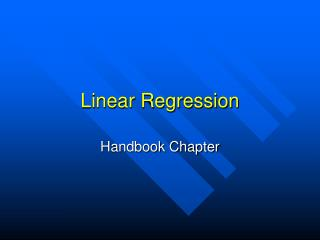DownloadDownload PresentationLinear Regression

Linear Regression

Télécharger la présentationLinear Regression

- - - - - - - - - - - - - - - - - - - - - - - - - - - E N D - - - - - - - - - - - - - - - - - - - - - - - - - - -
Presentation Transcript

1. Linear Regression Handbook Chapter

2. Experimental Testing • Data are collected, in scientific experiments, to test the relationship between various measurable quantities that are predicted by a hypothesis, either to support or invalidate the hypothesis. • The dependent variable is measured under varying, but preset, values of the independent variable.

3. Model Fitting • Correlation = • If the variables have a correlation, it is convenient to express the relationship in the form of a mathematical equation, known as a model or natural law. • The mathematical equation describes a functional relationship between the variables.

4. Model Fitting • It may be relatively simple to demonstrate the functional relationship between the dependent and independent variables if the relationship is direct. • Direct relationships (linear relationships) can be represented by a straight line.

5. Linear Models • A straight line is simplest statistically analyzable function. • One way to express the linear relationship between an independent variable (x) and a dependent variable (y) is the slope –intercept formula: y = m x + b.

6. Slope –Intercept Formula y = m x + b y = dependent variable x = independent variable m = slope of the line b = y-intercept of the line

7. Slope m = slope of the line • The slope represents the amount of tilt (slant) the straight line has relative to the x- and y- axes.

8. y – Intercept b = y-intercept of the line • The y-intercept is the point at which a straight line intersects the y-axis.

9. Slope –Intercept Formula • If the slope and y –intercept of a straight line is given, then the y value can be calculated, with confidence, for every given value of x. • The points of the straight line have the coordinates corresponding to the (x, y) pairs.

10. Linear Regression • Since measurements inherently contain both systematic and random errors, data points will not fit a given equation perfectly. • Linear regression is a statistical method for finding the best fitting straight line to a set of (x, y) pairs.

11. The expression giving the most probable slope of the fitted straight line is: NN N S xi2 –[S xi ]2 i=1 i=1 NNN N S yixi– S yi S xi i=1 i=1 i=1 m =

12. The expression giving the most probable y-intercept of the fitted straight line is: NN N S xi2 –[S xi ]2 i=1 i=1 NNN N S xi2S yi–S xiS yixii=1 i=1 i=1 i=1 b =